# Software/dSim

## dSim - Diffusion Simulator

The diffusion simulator (dSim) is a software program that simulates DTI measurements in the human brain by simulating the behavior of hydrogen particles within the brain structure.

The program is open source and can be checked out of its svn repository using the following command:

 svn co https://white.stanford.edu/repos/dSim/trunk/


### Software concept

The idea behind dSim is to allow the user to conduct simulations of DTI measurements on a user-defined structure of brain fibers and see whether the resulting data matches actual measurements. This allows for the testing of various hypotheses regarding fiber structure.

The user creates a small volume, typically with dimensions of around 0.1mm, and uploads a fiber structure into the volume, as shown in the image.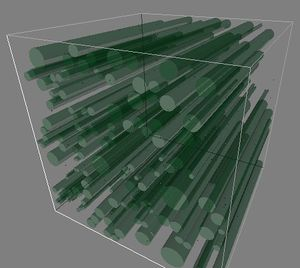The volume is then populated with water molecules, typically in the tens of thousands, which start moving in a Brownian motion upon the execution of the program. In the meantime, a sequence of magnetic gradient fields is applied to the volume and the resulting MRI measurements are recorded. The set of measurements are then used to compute the diffusivity parameters for the volume and to draw the corresponding diffusion ellipsoid.

Various parameters related to the fiber structure can be changed by the user, such as the degree of myelination, how easily particles diffuse, the T2 relaxation values of axons and myelin and many others.

### Theory of diffusion tensor imaging

The following sections give an overview of the theory behind DTI imaging. The more mathematical parts assume the reader has a basic understanding of magnetic resonance imaging, as well as understanding of calculus.

#### Intuitive approach

The human body contains a vast quantity of hydrogen atoms. When subjected to an external magnetic field, the magnetic moments of the hydrogen atoms align into what is commonly viewed as a magnetization vector in the same direction as the main field. By applying a certain type of electromagnetic pulse to the volume containing the atoms, the magnetization vector can be rotated, or "flipped", away from its original axis, which is called the z-axis in the usual notation. In the case of a 90 degree pulse, the magnetization is rotated 90 degrees and ends up perpendicular to the z-axis. The magnetization is then completely "transverse", as opposed to being "longitudinal". The transverse magnetization precesses about the z-axis with a precession frequency $\omega = \gamma B$ (in radians per second), which is proportional to the strength of the field. By applying a magnetic field gradient in addition to the main magnetic field, one can therefore make the field strength, and then the precession frequency as well, dependent on spatial location.

Imagine a magnetization vector at a particular point in space, subject to a magnetic gradient. If the gradient is applied for $\delta$ seconds, the magnetization will have rotated over a bigger angle than it would have if no gradient had been applied - it will have gained positive phase. If we then apply an identical gradient but with opposite sign (or, equivalently, if we apply a 180 degree flip and then an identical gradient), the magnetization will likewise gain negative phase and the net phase change in the end will be zero. We can think of this as the latter half of the gradient unwinding the phase gain from the first half, or as "rephasing" the magnetization.

Now imagine that the hydrogen particles are not stationary but moving randomly about. Then the effects of the two gradients will not be identical, and the magnetization at the end will (most likely) not have zero phase gain - the rephasing is imperfect. These imperfections are more apparent with increased diffusion, and also with longer or stronger gradients. In fact, as is shown below, the effectiveness of the rephasing depends on the quantity $e^{-bD}$, where b (called the b-value of the gradient) gets bigger with the strength and duration of the gradient, and D (called the diffusion constant of the particles) is proportional to the variance of the particle movement (the more the particles randomly move, the greater the value of D).

One can make use of this rephasing ineffectiveness to estimate how easily the particles diffuse, i.e. to estimate D. By applying gradient sequences like the one described along a number of direction, one get an estimate of the diffusivity in each gradient direction. The worse we do with the dephasing, the more the molecules must be diffusing in that directions. Usually, the minimum number of gradient orientations is 6, since this allows us to construct an ellipsoid, oriented along the principal diffusion direction, with axis lengths representing the diffusivity along the direction of each axis.

#### Mathematical approach

This section is heavily based on chapter 1 of Diffusion and Perfusion Magnetic Resonance Imaging, written by Denis Le Bihan and Peter J. Basser. The last section, on constructing the diffusion matrix from the measured echoes, is based on this webpage, written by Maj Hedehus and Stefan Skare.

##### Simple case: Short gradients in one dimension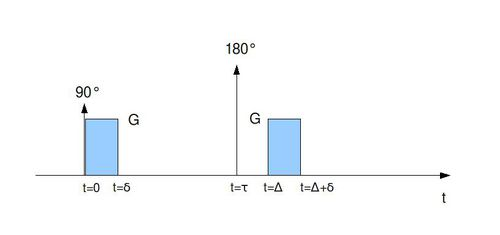Let us begin by assuming that the spins are only free to move along the z axis. Consider the gradient sequence shown in the image above. The durations of the gradients are $\delta$ and the time from the start of the first gradient to the start of the second is $\Delta$. Before the first gradient is applied, a 90 degree pulse flips the magnetization vector into the transverse plane. Before the application of the second gradient, a 180 degree pulse at time $\tau$ flips the magnetization in the transverse plane, resulting in an echo at time $2\tau$. We assume that the gradients are so short that the effects of diffusion are much more pronounced in the period between gradients than during the gradients (i.e. $\Delta >> \delta$). Now, a spin located at z while the gradient G is being applied will rotate at the frequency $\frac{\gamma}{2 \pi}Gz$, where $\gamma$ is the gyromagnetic ratio and the phase shift experienced during the first gradient will therefore be
$\phi_1 = \int_0^{\delta}Gz_1 {dt} = \gamma G \delta z_1$,
where $z_1$ is the location of the spin during the first gradient (we recall that we are assuming practically all diffusion to take place in the time between gradients, i.e. that the spins are stationary during the gradients). Likewise, the phase shift from the second pulse is $\phi_2 = -\gamma G \delta z_2$ (the minus sign is due to the 180 degree pulse). Thus, the total phase change will be
$\phi_{12} = \phi_1 + \phi_2 = \gamma G \delta (z_1 - z_2) = \gamma G \delta z_{12}$.
If the magnetization vector of each spin has magnitude $s_0$, we can therefore write the magnetization of spin j at the echo time as
$s_j = s_0 e^{i \phi_{12-j}}$
and the total magnetization at the echo time is therefore
$S = \sum_{j=1}^N s_0 e^{i \phi_{12-j}}$.
If no gradient is applied, we have $\phi_{12} = 0$ and therefore $S = S_0 = Ns_0.$ With a nonzero gradient, the signal relative to $S_0$ can then be written as $\frac{S}{S_0} = \frac{1}{Ns_0} \sum_{j=1}^N s_0 e^{i \phi_{12-j}} = \frac{1}{N} \sum_{j=1}^N e^{i \gamma G \delta z_{12-j}} = E(e^{i \gamma G \delta z_{12}})$.
If the spins move with Brownian motion with diffusion coefficient D, $z_{12}$, the distance the spin moves in a time period of length $\Delta$ is a normally distributed random variable with a variance of $2 D \Delta$ and therefore has a probability density function of
$f_{\Delta}(z_{12}) = \frac{1}{\sqrt{4 \pi D \Delta}}e^{-\frac{(z_{12})^2}{4 D \Delta}}$
and the relative signal can then be calculated as
$\frac{S}{S_0} = E(e^{i \gamma G \delta z_{12}}) = \frac{1}{\sqrt{4 \pi D \Delta}}\int_{-\infty}^{\infty} e^{i \gamma G \delta z_{12}} e^{-\frac{(z_{12})^2}{4 D \Delta}} dz_{12}$ $= \frac{1}{\sqrt{4 \pi D \Delta}} e^{-(\gamma G \delta)^2 D \Delta}\int_{-\infty}^{\infty} e^{-\frac{1}{4 D \Delta} (u - i\gamma 2 D \Delta \delta G)^2} du$ $= \frac{1}{\sqrt{4 \pi D \Delta}} e^{-(\gamma G \delta)^2 D \Delta}\int_{-\infty}^{\infty} e^{-q^2} 2\sqrt{D \Delta} dq = e^{-(\gamma G \delta)^2 D \Delta}.$

By substituting $b = (\gamma G \delta)^2 \Delta$ we therefore have
$\frac{S}{S_0} = e^{-bD} \Rightarrow D = -\frac{1}{b} (\log(S) - \log(S_0)).$
So, by taking a measurement with a gradient applied, comparing it with a measurement without gradients, and making use of the b-value, one can estimate the diffusivity.

When $\delta << \Delta$ does not apply, as shown in the figure above, it is useful to start by reviewing the familiar Bloch equations:
$\frac{\partial \bold{M}}{\partial t} = \gamma \bold{M} \times \bold{B} - \begin{bmatrix} \frac{1}{T_2} & 0 & 0 \\ 0 & \frac{1}{T_2} & 0 \\ 0 & 0 & \frac{1}{T_1} \end{bmatrix} \bold{M} + M_0 \begin{bmatrix} 0 \\ 0 \\ \frac{1}{T_1} \end{bmatrix}.$
If $B_x = B_y = 0$ this leads to
$\begin{bmatrix} \frac{\partial M_x}{\partial t} \\ \frac{\partial M_y}{\partial t} \\ \frac{\partial M_z}{\partial t} \end{bmatrix} = \begin{bmatrix} -\frac{1}{T_2} & \gamma B_z & 0 \\ -\gamma B_z & -\frac{1}{T_2} & 0 \\ 0 & 0 & -\frac{1}{T_1} \end{bmatrix} = \begin{bmatrix} M_x \\ M_y \\ M_z \end{bmatrix} + M_0 \begin{bmatrix} 0 \\ 0 \\ \frac{1}{T_1} \end{bmatrix}.$

By defining the transverse magnetization $M = M_x + iM_y$ we obtain
$\frac{dM}{dt} = \frac{dM_x}{dt} + i\frac{dM_y}{dt} = - \left( \frac{1}{T_2} + i \gamma B_z \right) M$
$\Rightarrow M = M_0 e^{-\left( \frac{1}{T_2} + i \gamma B_z \right) t}.$

If $B_z = B_0 + zG(t)$, i.e. the magnetic field consists of a static field and a time-varying gradient in the z-direction, then the solution can be written as
$M = M_0 e^{-\left( \frac{1}{T_2} + i \gamma B_0 \right) t} e^{-i \gamma z \int_0^t G(\tau) d\tau} = M_0 e^{-\left( \frac{1}{T_2} + i \omega_0 \right) t} e^{-i z k(t)},$
where $k(t) = \gamma \int_0^t G(\tau) d\tau.$

The above equations do not include diffusion. The diffusion of a particle concentration C along the z-axis can be described mathematically as $\frac{\partial C}{\partial z} = \frac{\partial}{\partial z} \left( D \frac{\partial C}{\partial z} \right),$ where D is the diffusion coefficient. Since the magnetization is proportional to the spin concentration, and we assume the diffusion coefficient to be independent of position in the volume being examined, the differential equation for the transverse magnetization can be written as
$\frac{dM}{dt} = - \left( \frac{1}{T_2} + i \gamma B_z \right) M + D \frac{\partial^2 M}{\partial z^2} = - \left( \frac{1}{T_2} + i \gamma B_0 \right) M - i \gamma z G(t) + D \frac{\partial^2 M}{\partial z^2}$
$\Rightarrow M(z,t) = M_0 e^{-\left( \frac{1}{T_2} + i \omega_0 \right) t} e^{-i \gamma z \int_0^t G(\tau) d\tau} e^{-D \int_0^t \gamma^2 \left( \int_0^{s} G(\tau) d\tau \right)^2 ds}$ $= M_0 e^{-\left( \frac{1}{T_2} + i \omega_0 \right) t} e^{-i z k(t)} e^{-D \int_0^t k^2(s) ds}.$

The magnetization at the echo time TE then is
$M(z,TE) = M(TE) = M_0 e^{-\left( \frac{1}{T_2} + i \omega_0 \right) TE} e^{-i z k(TE)} e^{-D \int_0^{TE} k^2(s) ds} = M_0 e^{-\left( \frac{1}{T_2} + i \omega_0 \right) TE}e^{-D \int_0^{TE} k^2(s) ds},$
where we have used that $k(TE) = \int_0^{TE} G(\tau) d\tau = 0$ because of the equal areas of the two parts of the gradient and the application of the 180 degree pulse. Therefore, the ratio between the echoes with and without gradients is
$\frac{M(TE)}{M_{G=0}(TE)} = e^{-D \int_0^{TE} k^2(s)ds} = e^{-bD},$ where
$b = \int_0^{TE} k^2(s) ds = \int_0^{\delta} (\gamma G s)^2 ds + \int_{\delta}^{\Delta} (\gamma G \delta)^2 ds + \int_{\Delta}^{\Delta+\delta} (\gamma G (\delta - (s-\Delta)))^2 ds$ $= \frac{1}{3} \gamma^2 G^2 \delta^3 + \gamma^2 G^2 \delta^2(\Delta-\delta) + \frac{1}{3} \gamma^2 G^2 \delta^3 = (\gamma G \delta)^2 \left( \Delta - \frac{\delta}{3} \right).$
We note that when $\delta << \Delta,$ the solution reduces to the answer in the simple case above.

##### General case: Anisotropic diffusion in three dimensions

If the spins can move in three dimensions, we denote the spin positions, gradient and diffusivity as
$\bold{r} = \begin{bmatrix} x \\ y \\ z \end{bmatrix}, \bold{G} = \begin{bmatrix} G_x \\ G_y \\ G_z \end{bmatrix}, \bold{D} = \begin{bmatrix} D_{xx} & D_{xy} & D_{xz} \\ D_{xy} & D_{yy} & D_{yz} \\ D_{xz} & D_{yz} & D_{zz} \\ \end{bmatrix}.$
In the matrix $D$, the diagonal terms $D_{xx}$, $D_{yy}$ and $D_{zz}$ reflect the variance of molecular movement in the x, y and z directions, while the off-diagonal elements reflect correlations between movement in orthogonal directions.
The solution to the differential equation governing the magnetization behavior then becomes
$M(\bold{r},t) = M_0 e^{-\left( \frac{1}{T_2} + i \omega_0 \right) t} e^{-i \bold{r \cdot k}(t)} e^{- \int_0^t \bold{k}(s)^T \bold{D} \bold{k}(s) ds},$
where $\bold{k}(t) = \gamma \int_0^t \bold{G}(t') dt' = \gamma \begin{bmatrix} \int_0^t G_x(t') dt' \\ \int_0^t G_y(t') dt' \\ \int_0^t G_z(t') dt' \\ \end{bmatrix}.$
In the case of isotropic diffusion we get
$\bold{D} = \begin{bmatrix} D & 0 & 0 \\ 0 & D & 0 \\ 0 & 0 & D \\ \end{bmatrix}$.
With anisotropic diffusion, we can define a b-matrix
$\bold{b} = \int_0^t \bold{k}(s) \bold{\cdot} \bold{k}(s) ds = \begin{bmatrix} b_{xx} & b_{xy} & b_{xz} \\ b_{xy} & b_{yy} & b_{yz} \\ b_{xz} & b_{yz} & b_{zz} \\ \end{bmatrix}$
where $b_{ij} = \int_0^t \gamma^2 \left( \int_0^uG_i(\tau) d\tau \right) \left( \int_0^uG_j(\tau) d\tau \right) du$
and we can then write $\frac{M(TE)}{M_{G=0}(TE)} = e^{-\sum_{i=x,y,z} \sum_{j=x,y,z} b_{ij} D_{ij}}$.

Let us now examine what happens if our gradient is of the form $\bold{G} = \begin{bmatrix} G \\ G \\ 0 \\ \end{bmatrix}$.

Then $b_{xx} = b_{yy} = b_{xy} = b_{yx} = (\gamma G \delta)^2 \left( \Delta - \frac{\delta}{3} \right) = b$ as before, and $b_{ij} = 0$ for all other values of i and j. We then get, by defining $M_{\mathbf{G} = [G,G,0]^T}(TE) = S_{110}$ and $M_{G=0}(TE) = S_0$ for clarity,
$\frac{S_{110}}{S_0} = e^{-b(D_{xx}+D_{xx}+2D_{xy})}$,
where we have used that $D_{xy} = D_{yx}$. We therefore get
$\frac{-1}{b} \ln{ \left( \frac{S_{110}}{S_0} \right)} = D_{xx}+D_{yy}+2D_{xy}$.
By doing similar acquisitions with different combinations of gradient components we get
$\frac{-1}{b} \begin{bmatrix} \ln{ \left( \frac{S_{110}}{S_0} \right)} \\ \ln{ \left( \frac{S_{101}}{S_0} \right)} \\ \ln{ \left( \frac{S_{011}}{S_0} \right)} \\ \ln{ \left( \frac{S_{-110}}{S_0} \right)} \\ \ln{ \left( \frac{S_{-101}}{S_0} \right)} \\ \ln{ \left( \frac{S_{0-11}}{S_0} \right)} \\ \end{bmatrix} = \begin{bmatrix} 1 & 1 & 0 & 2 & 0 & 0 \\ 1 & 0 & 1 & 0 & 2 & 0 \\ 0 & 1 & 1 & 0 & 0 & 2 \\ 1 & 0 & 0 & -2 & 0 & 0 \\ 1 & 0 & 1 & 0 & -2 & 0 \\ 0 & 1 & 1 & 0 & 0 & -2 \\ \end{bmatrix} \begin{bmatrix} D_{xx} \\ D_{yy} \\ D_{zz} \\ D_{xy} \\ D_{xz} \\ D_{yz} \\ \end{bmatrix}$.
The gradient sequence shown above is known as a dual-echo scheme and is commonly used in DTI imaging. We can then retrieve the diffusion coefficients by solving the above matrix equation:
$\begin{bmatrix} D_{xx} \\ D_{yy} \\ D_{zz} \\ D_{xy} \\ D_{xz} \\ D_{yz} \\ \end{bmatrix} = \frac{-1}{b} \begin{bmatrix} 1 & 1 & 0 & 2 & 0 & 0 \\ 1 & 0 & 1 & 0 & 2 & 0 \\ 0 & 1 & 1 & 0 & 0 & 2 \\ 1 & 0 & 0 & -2 & 0 & 0 \\ 1 & 0 & 1 & 0 & -2 & 0 \\ 0 & 1 & 1 & 0 & 0 & -2 \\ \end{bmatrix} ^{-1} \begin{bmatrix} \ln{ \left( \frac{S_{110}}{S_0} \right)} \\ \ln{ \left( \frac{S_{101}}{S_0} \right)} \\ \ln{ \left( \frac{S_{011}}{S_0} \right)} \\ \ln{ \left( \frac{S_{-110}}{S_0} \right)} \\ \ln{ \left( \frac{S_{-101}}{S_0} \right)} \\ \ln{ \left( \frac{S_{0-11}}{S_0} \right)} \\ \end{bmatrix}$.
If a gradient sequence with a different number of gradients is used, resulting in a non-square matrix, then a pseudo-inverse should be used. The code used in dSim to evaluate the matrix inverse makes use of the Template Numerical Toolkit (TNT) and the Jama Linear Algebra Package, developed by the National Institute of Standards and Technology and available here.
This way, we can estimate all the coefficients of our diffusion tensor, $\bold{D} = \begin{bmatrix} D_{xx} & D_{xy} & D_{xz} \\ D_{xy} & D_{yy} & D_{yz} \\ D_{xz} & D_{yz} & D_{zz} \\ \end{bmatrix},$
which should give us a complete description of the diffusion properties of our volume. It is often useful to visualize this tensor as an ellipsoid in 3D space. This can be achieved by computing the eigenvalues of the tensor and the corresponding eigenvectors and then constructing an ellipsoid with major, medium and minor axes oriented as the major, medium and minor eigenvectors, respectively, and having sizes equal to the respective eigenvalues.

### Methods and calculations

#### Fiber structure

The simulation volume can be thought of as composed of a number of compartments. The compartments can be of 4 different types; axons, the myelin sheaths surrounding the axons, glial cells and the space between fibers. In the program code, these compartment types are numbered as compartment type 0, 1, 2 and 3, respectively. The tissue properties (diffusion constant and T2) depend on the compartment type. Separating these compartments are three different types of membranes. When the program is given a description of the fiber structure, as is further discussed in the section on using dSim, the user must specify the type of membrane the triangles belong to. The compartment/membrane concepts are illustrated in the figure below.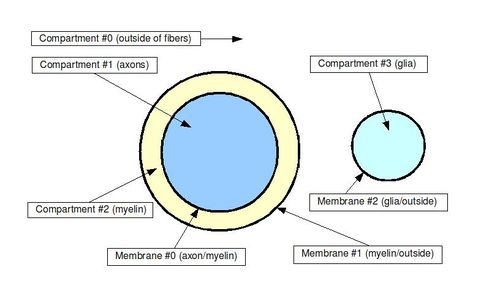#### Particle movement

At the beginning of program execution, the particles are uniformly randomly distributed throughout the volume. Some particles will be located inside the axons themselves, some will be in the myelin sheaths surrounding the axons and the rest will be in between fibers. This is often referred to as the particle being located in a particular compartment. The diffusion constant (how easily the particle diffuses) and the T2 relaxation constant depend on the compartment type the particle is in.

The program executes a series of time steps until all the magnetic gradient fields have been applied. The size of each timestep can be set by the user. During each timestep, each particle moves with a gaussian random walk in the x, y and z directions, i.e. for each direction, each spin experiences a displacement drawn from a normal distribution with mean 0 and standard deviation $\sqrt{2 \cdot ADC \cdot \Delta t}$, where ADC is the apparent diffusion constant (which depends on the compartment the particle is in) and $\Delta t$ is the length of the time step. The mean squared displacement of the random walk over all direction should then be $6 \cdot ADC \cdot \Delta t$ and with a small enough time step, this should result in approximately Brownian motion (see here for further information on random walks).

For generating the random numbers, we use a multiply-with-carry algorithm by George Marsaglia, which uses 2 32-bit seeds and has period greater than 2^60. This gives us a uniformly distributed random variable which is subsequently converted to a normal random variable using the Box-Muller transform in basic form.

Every time a particle moves in such a manner, the program checks whether the particle collides with a fiber membrane, in which case it either bounces off the membrane with probability (1-p) or permeates into the fiber with probability p (p is normally very small, on the order of 1e-6).

The image below shows examples of particles paths inside an axon (D = 2.1 $\mu m^2/s$, red color), in the myelin sheath (D = 0.1 $\mu m^2/s$, green color) and outside (D = 2.1 $\mu m^2/s$, blue color) of a fiber with outer radius 2.7 $\mu m$ and a g-ratio of 0.8.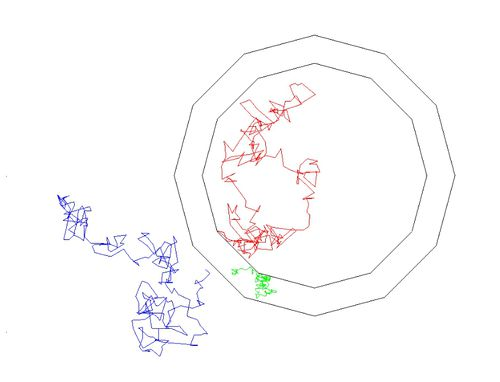#### Collision detection

The fiber membranes consist of triangle meshes, which are read into the simulator through a fiber file specified in the configuration file normally called sim.cfg. For each particle during each timestep, the program searches for triangles which the particle possibly collides with. This is done using one of two collision detection methods (the choice of method is made by the user); A uniform rectangular grid or an R-Tree.

The rectangular grid method divides the volume up into a set of cubes, the number of which depends on the maximum triangle size used in the fiber structure. For each particle, the program then loops through all the triangles in the same cube as the particle path and determines whether there is a collision. If the particle travels through more than one cube, the cubes that the particle starts and ends in are examined.

The R-Tree method creates an R-Tree, where each leaf contains a bounding rectangle of a triangle, and determines whether the bounding rectangle of the particle path intersects any of the leaf rectangles by traversing the tree. Our implementation of the R-Tree is a slightly altered version of a C++ header file written by Greg Douglas which can be obtained here.

The speed difference between the two methods depends on the fiber structure being simulated but the R-Tree is generally preferable to the grid.

#### Signal measurement

The method for estimating the MR signal is a follows: After each time step, spin number $i$ has a position vector $\bold{r_i}$. It will experience a phase shift of

$\Delta \phi = 2 \pi g \Delta t \bold{G} \cdot \bold{r_i}$

where $\bold{G}$ is the magnetic gradient, $\Delta t$ is the time step and $g = \frac{\gamma}{2 \pi}$ (= 42.576 MHz/T), where $\gamma$ is the gyromagnetic ratio.
Furthermore, if we look at each spin as representative of a larger pool of spins at that same location, then the pool will experience T2 relaxation according to $S_i(t) = S_i(0) e^{-t/T_2}$, where $T_2$ depends on the compartment the spins are in.

By summing the signal contributions from all N spins, we then obtain the total signal

$S(t) = \frac{\sqrt{\left( \sum S_i \cos\phi_i \right)^2 + \left( \sum S_i \sin\phi_i \right)^2}}{N}$.

### Explanation of code

The source code mainly consists of the files diffusion.cpp, spinSystem.cpp, spinKernelCpu.cpp, spinSystem.cu and spinKernel.cu.

diffusion.cpp contains the main function which is run at program execution. This code reads the simulation parameters, creates a spin system, controls the graphical display of the simulation if requested, applies the magnetic gradient sequence to the spin system, computes the diffusion parameters from the resulting magnetic resonance measurements and writes the results to an output file.

spinSystem.cpp contains the definition of the spin system class, an object of which is created during the run of diffusion.cpp. The methods of the class include reading the brain fiber structure, creating a rectangular grid or R-Tree for collision detection, reading the MR signal of the system, determining which compartment type each particle belongs to and updating the position of the spins.

spinSystem.cu is called from spinSystem.cpp when the computation is to be performed on the GPU. It contains functions for copying data between host and device and for executing the parallel spin calculations implemented in spinKernel.cu.

spinKernel.cu contains the methods used in updating the position and signal of each particle, including methods for collision detection. This is performed in parallel for each thread in the computation (the number of which is set in spinSystem.cu).

spinKernelCpu.cpp performs a similar function as spinKernel.cu, but on the CPU instead of the GPU. This is done for consistency between the two sets of code.

The below image further illustrates the structure of the code.

### Using dSim

dSim is designed for a Linux operating system (developed on Ubuntu 9.04). Furthermore, to run the program on the GPU for greatly increased speed, a CUDA compatible GPU card is needed.

#### Installing CUDA

In order to compile the dSim program, the CUDA Software Development Kit (SDK) from NVIDIA needs to be installed, as well as the SDK code samples on NVIDIA's website. Assuming the user is running a 64-bit version of Ubuntu, the installation steps are as follows:

##### Installing the CUDA toolkit

The CUDA toolkit can be obtained by going to the NVIDIA website, choosing "technologies" in the menu at the top, moving the pointer to "CUDA technology" on the left side of the page, clicking "Download CUDA SDK" and then following the link to the latest release (or equivalently going directly to http://developer.nvidia.com/cuda-toolkit-32-downloads for version 3.2, which is the current one as of this writing). Choose the link to the 64-bit CUDA toolkit for Ubuntu. Your browser should download a .run file to your hard drive. Open up a terminal, cd to the directory containing the file and run it by typing "sudo sh" followed by the name of the file, then hit enter. An installer will run, asking the user where the toolkit should be installed. The default location (/usr/local/cuda) is appropriate. Upon the completion of the installation, the user should be able to compile basic CUDA programs. However, the locations of the necessary binaries and libraries need to be available to the compiler via the PATH and LD_LIBRARY_PATH environment variables. This can be done by typing "PATH=$PATH:/usr/local/cuda/bin" and "LD_LIBRARY_PATH=$LD_LIBRARY_PATH:/usr/local/cuda/lib64". Note that these changes only apply to the current shell and will need to be reset if a new shell is opened. In order to set these variables permanently, the export commands stated above can be added at the bottom of the .bashrc file located in the user's home directory (i.e. ~/.bashrc). Note: A bug exists in some versions of Ubuntu where a conflict between ssh-agent and the running of .profile results in the nulling of LD_LIBRARY_PATH at startup. This can be solved by changing use-ssh-agent in the file /etc/X11/Xsession.options to no-use-ssh-agent. This issue appears to have been fixed in more recent versions of Ubuntu.

##### Installing SDK code samples

In theory, the CUDA toolkit provides everything necessary to write and compile CUDA programs. However, dSim makes use of certain extra tools that come with the code samples on the NVIDIA site, specifically the cutil library, which makes certain data handling easier. In order to install the SDK samples, go to the same download site as for the CUDA toolkit, click the link "GPU Computing SDK code samples" and run the installation file using the sh command as before. The installer will ask you were to install the samples. Please note that while the user is free to place the code samples anywhere on the hard drive, dSim assumes that they are installed under /usr/local/cuda/sdk (which is not the default installation directory). If another location is chosen, the dSim Makefile must be changed accordingly. In these installation instructions, the user is assumed to have chosen that directory for installation. To make sure the libraries included with the code samples can be found by the system, add /usr/local/cuda/sdk/C/lib to LD_LIBRARY_PATH (see instructions above). Once the code samples have been installed and the library path has been updated, they can be compiled by going to /usr/local/cuda/sdk and typing make. If everything compiles, the binaries should be located in /usr/local/cuda/sdk/C/bin/linux/release. The user is encouraged to try some of them out. If everything works out, dSim can then be installed.

##### Updating NVIDIA driver

In some instances, the graphics card driver may be too old to run CUDA programs properly. This can be solved by installing a new driver. To be continued...

##### Troubleshooting

When trying to compile the SDK code samples, I get the error message "/usr/bin/ld: cannot find -lXi".
The system does not have the Xi development library, which is used by some of the SDK code samples to produce GUIs using the X windows system. Go to System->Administration->Synaptic Package Manager and choose the libxi-dev package.

When trying to compile the SDK code samples, I get the error message "/usr/bin/ld: cannot find -lXmu".
This is similar to the error above. The system does not have the Xmu development library, which is used by some of the SDK code samples for X windows functionality. Go to System->Administration->Synaptic Package Manager and choose the libxmu-dev package.

When trying to compile the SDK code samples, I get the error message "/usr/bin/ld: cannot find -lGL".
Some of the SDK code samples use the OpenGL framework for displaying graphics. This is fixed by going to System->Administration->Synaptic Package Manager and choose the libglut3-dev package.

When trying to compile the SDK code samples, I get the error message "/usr/bin/ld: cannot find -lGLEW".
This is similar to the last error, some of the code samples need the OpenGL Extension Wrangler (GLEW) library. This is fixed by going to System->Administration->Synaptic Package Manager and choose the libglew1.5-dev package.

When trying to compile the SDK code samples, I get the error message "/usr/bin/ld: cannot find -lcuda".
This could mean that your graphics card driver does not support CUDA. See above instructions for updating your driver.

When trying to compile the SDK code samples, I get the error message "error while loading shared libraries: libcudart.so.3: cannot open shared object file: No such file or directory".
The compiler cannot find the CUDA run time library, cudart. Make sure that your library path LD_LIBRARY_PATH contains /usr/local/cuda/lib64

#### Installing dSim

Start by checking out the code from the repository using the svn command

svn co https://white.stanford.edu/repos/dSim/dSim3


This should install the source files along with the executable file to run the code. To run the program, simply type ./bin/release/diffusion while in the dSim directory. For recompiling the code, type "make" while in the dSim directory, preferably doing a "make clean" command first.

#### Running dSim

Configuration File: When the executable file is run by typing "./bin/release/diffusion", it will try to read a configuration file named "sim.cfg". The format of the configuration file should be as shown below:

app: {					// Parameters that control the graphical display of the diffusion process
stepsPerUpdate = 10;		// Number of timesteps per display update.
useGpu = true;			// If true, spin movement and signal calculations are performed on the GPU. Otherwise, they are performed on the CPU.
useDisplay = true;		// If true, the fibers and spins are rendered on the computer screen. Results in some speed reduction.
writeOut = true;                // If true, the output will be written to the outFile specified below.
};

sim: {					// Parameters for diffusion calculations
numSpins = 30000;		// The number of spins simulated
gyroMagneticRatio = 42576.0;	// Gyromagnetic ratio / (2 pi). Units: (ms*T)^(-1)
timeStep = 0.005;		// Time passed during each computational step. Units: ms.
extraAdc = 2.1;			// Apparent diffusion constant outside of fibers. Units: um^2/ms.
intraAdc = 2.1;			// Apparent diffusion constant inside axons. Units: um^2/ms.
myelinAdc = 0.1;		// Apparent diffusion constant in myelin sheath. Units: um^2/ms.
extraT2 = 80.0;			// T2 relaxation time outside of fibers. Units: ms.
intraT2 = 80.0;			// T2 relaxation time inside axons. Units: ms.
myelinT2 = 7.5;			// T2 relaxation time in myelin sheath. Units: ms.
spaceScale = 100.0;		// The volume extends from -spaceScale to +spaceScale in all directions. Units: um.
permeability = 0.000001;	// Probability of spin permeating through membrane during collision.

outFile = "output.m";		// Name of file to which the program output is written
};

fibers: {
fiberFile = "sim.fibers";	// Name of file containing the triangle mesh which makes up the fiber structure.
};

## eof


Many of the parameters in the configuration file can also be specified by using the appropriate flag when running the program from the command line. If a parameter is specified both in the configuration file and at the command line, then the command line value has preference. The following flags are available:

-disp:             Display the simulation graphically on the monitor (overrides 'useDisplay' in the config file).
-nodisp:           Do not display the simulation graphically on the monitor (overrides 'useDisplay' in the config file).
-gpu:              Run the simulation using the GPU (overrides 'useGpu' in the config file).
-cpu:              Run the simulation entirely on the CPU (overrides 'useGpu' in the config file).
-w:                Write the results to the output file (see description below - overrides 'writeOut' in the config file).
-nw:               Do not write the results to the output file (see description below - overrides 'writeOut' in the config file).
-stepsPerUpdate=N: Set the number of steps per update to N (overrides 'stepsPerUpdate' in the config file).
-numSpins=N:       Set the number of spins to N (overrides 'numSpins' in the config file).
-perm=X:           Set the permeability probability to X (overrides 'permeability' in the config file).
-fiberFile="F":    Set the name of the file containing the fibers to F (overrides 'fiberFile' in the config file).


For example, the following command runs the program on the CPU, with 25,000 spins, and reads the fiber structure from the file 'fibers/sim.fibers01':

./bin/release/diffusion -cpu -numSpins=25000 -fiberFile="fibers/sim.fibers01"


Three more files are specified in the configuration file:

gradsFile: This file specifies the magnetic gradient sequence applied to the volume. The file format should be as shown below:

10.00 12.0669 1.00 0.0 0.0 0.0

10.00 12.0669 1.00 80.0 80.0 0.0

10.00 12.0669 1.00 -80.0 -80.0 0.0

10.00 12.0669 1.00 0.0 80.0 80.0

10.00 12.0669 1.00 0.0 -80.0 -80.0

10.00 12.0669 1.00 80.0 0.0 80.0

10.00 12.0669 1.00 -80.0 0.0 -80.0

10.00 12.0669 1.00 -80.0 80.0 0.0

10.00 12.0669 1.00 80.0 -80.0 0.0

10.00 12.0669 1.00 0.0 -80.0 80.0

10.00 12.0669 1.00 0.0 80.0 -80.0

10.00 12.0669 1.00 80.0 0.0 -80.0

10.00 12.0669 1.00 -80.0 0.0 80.0


Each line represents one gradient in the sequence. The first column is the length of the pulse (small delta, $\delta$), the second column is the time between pulses (big delta, $\Delta$) and the third column is the readout time. Units for all three parameters are milliseconds. Columns four, five and six represent the x, y and z components of the gradient, with units in mT/m.

outFile: The simulation output will be written to this file. The output data is designed to be easy to work with in Matlab, so a .m file extension is appropriate.

fiberFile: This file specifies the triangle mesh that makes up the fiber structure. The file format should be as shown below:

V
-0.08   0        -1
-0.08   0   -0.8571
-0.08   0   -0.7143
-0.08   0   -0.5714
-0.08   0   -0.4286
-0.08   0   -0.2857
T
0      1     2
1      2     3
2      3     4
3      4     5
A0
0
1
M0
2
3


Each line following the "V" represents the x, y and z coordinates of a vertex. Each line following the "T" represents the vertices of a triangle. Each line following "An" or "Mn" represents the triangles belonging to the axon or myelin sheath, respectively, of fiber n.

#### Generating fibers

There is currently not an official built-in module in dSim to generate a fiber file such as the one shown above. However, in the dSim repository there is a Matlab file, "matlab_tests/fiber_generation/fiberPlot.m", which takes as input the two endpoints and the middle point of each fiber, as well as its outer radius and g-ratio (the ratio between the axon radius and the myelin outer radius) and generates a triangle mesh for the fiber, for any number of fibers. No constraint is put on the fiber geometry, except that each membrane should be a closed surface (no holes in any membrane) and two membranes should not intersect.

### Sample results

#### Graphical ouput

When the simulation has finished running, the resulting diffusion ellipsoid is drawn (if the program is set to graphics mode) and parameters describing the diffusion (the diffusion tensor D, its three eigenvalues, mean diffusivity, fractional anisotropy and radial diffusivity) are written to the console. An example is shown below, with 107 fibers oriented along the z-axis.

#### Numerical data

To measure how mean diffusivity, radial diffusivity and fractional anisotropy depend on fiber thickness and myelination, these parameters were varied for a number of simulations and the output plotted in Matlab.

A simulation volume of spacescale 60 (size 120x120x120 $\mu m^3$) was made to contain NxN identical cylindrical fibers, each time filling the same fraction of the volume. The distance between adjacent fibers was 1/9 of the fiber diameter - one can think of this as dividing the cross section of the volume up into NxN boxes like the one shown below:

The volume fraction taken up by the fibers will be $\frac{0.81\pi}{4}$.

This was done for values of N from 15 to 25. For each such packing arrangement, the ratio between the outer fiber diameter and the inner diameter (i.e. the diameter of the axon excluding the myelin, which we call the g-ratio, was varied (by changing the inner diameter) from 0.5 to 0.9, with step size of 0.1.

The total number of fiber constructs was thus 11x5 = 55.

The resulting values of mean diffusivity, fractional anisotropy and radial diffusivity for the volume are shown in the images below.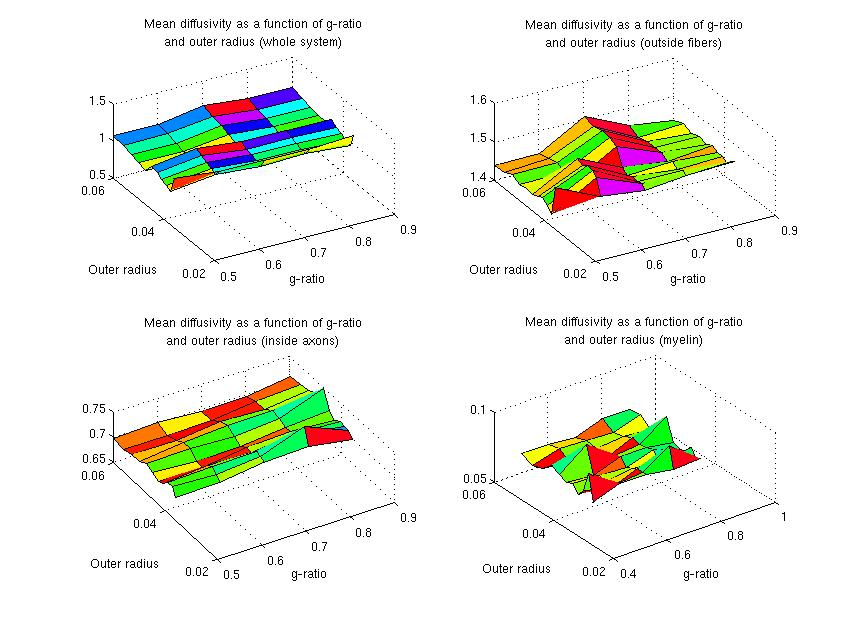3D plots of mean diffusivity as a function of outer radius and g-ratio.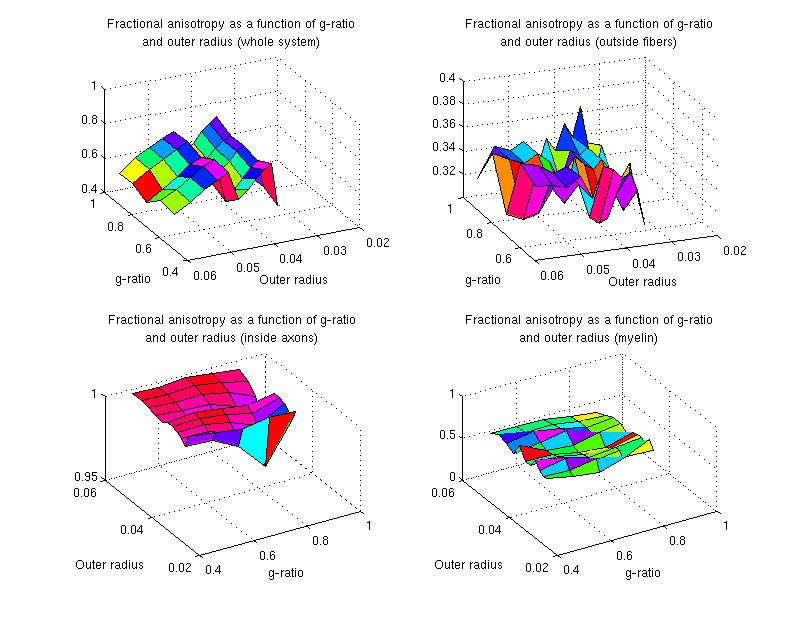3D plots of fractional anisotropy as a function of outer radius and g-ratio.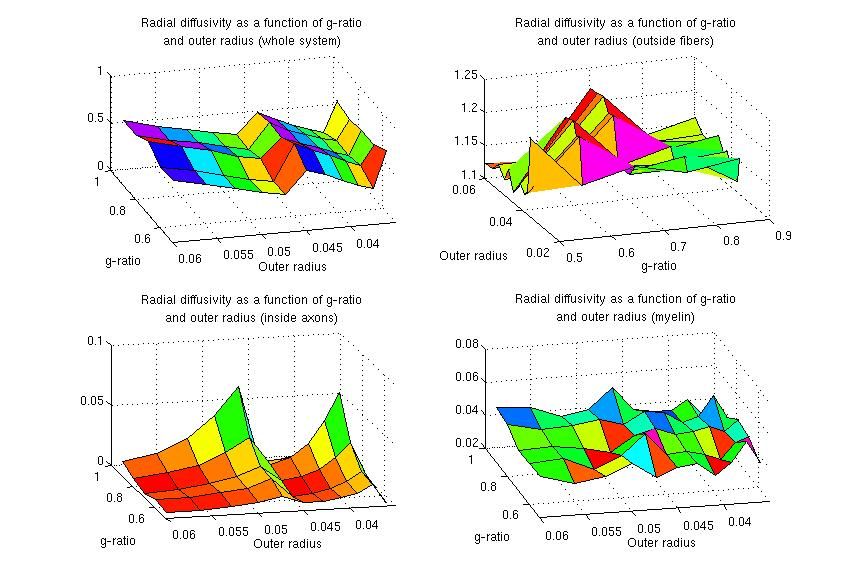3D plots of radial diffusivity as a function of outer radius and g-ratio.

The actual data values can be found in this zip file, which includes the results in Matlab m-file format (results.m), the simulation parameters (sim.cfg), the gradient file (sim.grads), the bash script used to run the program multiple times (bashRun.sh) and the fiber files (of format sim.fibers_[Number of fibers]_r[Outer radius]_f[g_ratio]).